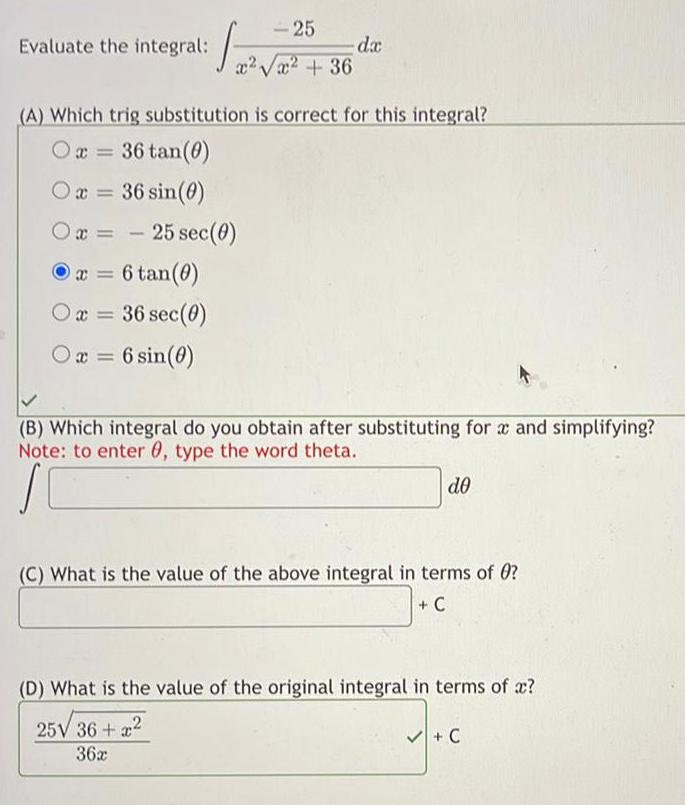Question:

# Evaluate the integral: ∫ -25 / (x²√(x²+36) dx (A) WhichEvaluate the integral: ∫ -25 / (x²√(x²+36) dx (A) Which trig substitution is correct for this integral? x= 36 tan(θ) x= 36 sin(θ) x= -25 sec (θ) x = 6 tan(θ) x = 36 sec (θ) x = 6 sin(θ) (B) Which integral do you obtain after substituting for x and simplifying? (C) What is the value of the above integral in terms of θ? (D) What is the value of the original integral in terms of x?